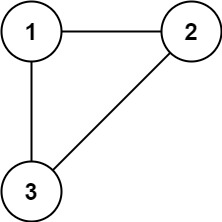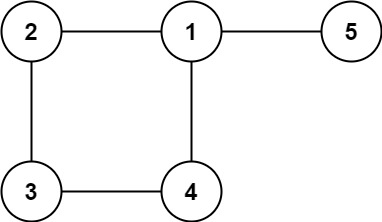# 684. Redundant Connection

Medium
In this problem, a tree is an undirected graph that is connected and has no cycles.
You are given a graph that started as a tree with `n` nodes labeled from `1` to `n`, with one additional edge added. The added edge has two different vertices chosen from `1` to `n`, and was not an edge that already existed. The graph is represented as an array `edges` of length `n` where `edges[i] = [ai, bi]` indicates that there is an edge between nodes `ai` and `bi` in the graph.
Return an edge that can be removed so that the resulting graph is a tree of `n` nodes. If there are multiple answers, return the answer that occurs last in the input.
Example 1:Input: edges = [[1,2],[1,3],[2,3]]
Output:
[2,3]
Example 2:Input: edges = [[1,2],[2,3],[3,4],[1,4],[1,5]]
Output:
[1,4]
Constraints:
• `n == edges.length`
• `3 <= n <= 1000`
• `edges[i].length == 2`
• `1 <= ai < bi <= edges.length`
• `ai != bi`
• There are no repeated edges.
• The given graph is connected.

### 解題

Runtime: 3 ms, faster than 93.75%
Memory Usage: 3.2 MB, less than 31.25%
func findRedundantConnection(edges [][]int) []int {
parent := make([]int, 0) // 紀錄源頭
for i := 0; i <= len(edges); i++ {
parent = append(parent, i)
}
for _, edge := range edges {
parent1 := find(edge, parent)
parent2 := find(edge, parent)
if parent1 == parent2 { // 已經有同一個源頭 = 早就相連
return edge
}
parent[parent2] = parent1 // 有相連的邊了，將不同源頭改為同一個
}
return []int{}
}
func find(n int, parent []int) int { // 找到源頭
if parent[n] == n {
return n
} else {
return find(parent[n], parent)
}
return 0
}